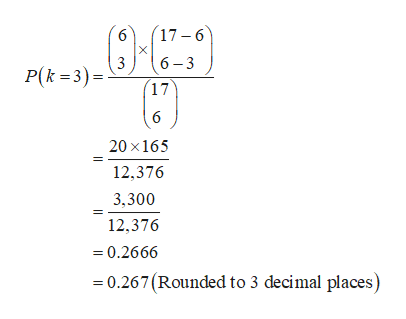# The state runs a lottery once every week in which six numbers are randomly selected from 17 without replacement. A player chooses six numbers before the state’s sample is selected. The player wins if all 6 numbers match. What is the probability that exactly 3 of the 6 numbers chosen by a player appear in the state’s sample? Report answer to 3 decimal places.

Question
24 views
The state runs a lottery once every week in which six numbers are randomly selected from 17 without replacement. A player chooses six numbers before the state’s sample is selected. The player wins if all 6 numbers match. What is the probability that exactly 3 of the 6 numbers chosen by a player appear in the state’s sample? Report answer to 3 decimal places.

check_circle

Step 1

Hyper geometric distribution:

A hyper geometric distribution is a discrete probability distribution that determines the probability of getting k successes in n draws (without replacement) from a finite population of size N that contains exactly K success states.

Denote the total number of successes as k,

Denote the total number of objects that are drawn without replacement as n,

Denote the population size as N,

Denote the total number of success states in the population as K.

The probability distribution of k is a hyper geometric distribution with parameters (N, K, n) and the probability mass function (pmf) of k is given as:

Step 2

Parameters of hyper geometric distribution for the given situation:

In a state’s lottery, 6 numbers are randomly selected from a total of 17 numbers without replacement.

Here, the population size is N =17,

The number of draws is n = 6.

Furthermore, it is given that, a player chooses 6 numbers before the state’s sample is selected and the player wins, if all the 6 numbers match with the state’s sample.

Thus, the number of success states in the population is K = 6.

The probability distribution of k successes is a hyper geometric distribution with parameters (N = 17, K = 6, n = 6) and the probability mass function (pmf) of k is given as:

Step 3

Probability that exactly 3 of the 6 numbers chosen by a player appear in the state’s sample:

It is given that, 3 of the 6 chosen numbers have to appear in the state’s lottery.

That is, the number of successes is ...help_outlineImage Transcriptionclose6 (17-6 X 3 6 3 (17 6 P(k 3) 20 x 165 12,376 3,300 12,376 =0.2666 0.267(Rounded to 3 decimal places) fullscreen

### Want to see the full answer?

See Solution

#### Want to see this answer and more?

Solutions are written by subject experts who are available 24/7. Questions are typically answered within 1 hour.*

See Solution
*Response times may vary by subject and question.
Tagged in

### Probability